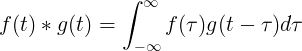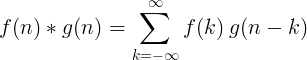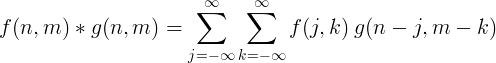# Convolution

Convolution is the correlation function of f(τ) with the reversed function g(t-τ).

The convolution operator is the asterisk symbol * .

### Continuous convolution

The convolution of f(t) and g(t) is equal to the integral of f(τ) times f(t-τ):### Discrete convolution

Convolution of 2 discrete functions is defined as:### 2D discrete convolution

2 dimensional discrete convolution is usually used for image processing.### Filter implementation with convolution

We can filter the discrete input signal x(n) by convolution with the impulse response h(n) to get the output signal y(n).

y(n) = x(n) * h(n)

### Convolution theorem

The Fourier transform of a multiplication of 2 functions is equal to the convolution of the Fourier transforms of each function:

ℱ{f  ⋅ g} = ℱ{f } * ℱ{g}

The Fourier transform of a convolution of 2 functions is equal to the multiplication of the Fourier transforms of each function:

ℱ{f  * g} = ℱ{f } ⋅ ℱ{g}

#### Convolution theorem for continuous Fourier transform

ℱ{f (t) ⋅ g(t)} = ℱ{f (t)} * ℱ{g(t)} = F(ω) * G(ω)

ℱ{f (t) * g(t)} = ℱ{f (t)} ⋅ ℱ{g(t)} = F(ω) ⋅ G(ω)

#### Convolution theorem for discrete Fourier transform

ℱ{f (n) ⋅ g(n)} = ℱ{f (n)} * ℱ{g(n)} = F(k) * G(k)

ℱ{f (n) * g(n)} = ℱ{f (n)} ⋅ ℱ{g(n)} = F(k) ⋅ G(k)

#### Convolution theorem for Laplace transform

ℒ{f (t) * g(t)} = ℒ{f (t)} ⋅ ℒ{g(t)} = F(s) ⋅ G(s)

Currently, we have around 1975 calculators, conversion tables and usefull online tools and software features for students, teaching and teachers, designers and simply for everyone.